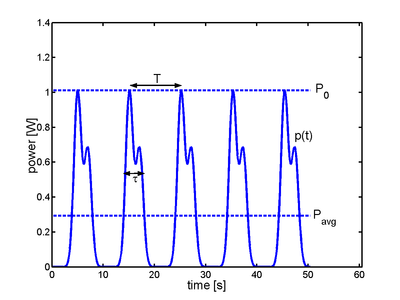In physics, power (symbol: P) is the amount of work done per unit of time.

Definition

This can be modeled as an energy flow, equivalent to the rate of change of the energy in a system, or the time rate of doing work,where

P is power
E is energy
W is work
t is time.

The average power (often simply called "power" when the context makes it clear) is the average amount of work done or energy transferred per unit time. The instantaneous power is then the limiting value of the average power as the time interval Δt approaches zero.When the rate of energy transfer or work is constant, all of this can be simplified towhere W and E are, respectively, the work done or energy transferred in time t.

Units

The units of power are units of energy divided by time. The SI unit of power is the watt, which is equal to one joule per second. The power consumption of a human  is on average roughly 100 watts, ranging from 85 W during sleep to 800 W or more while playing a strenuous sport. Professional cyclists have been measured at 2000 W output for short periods of time.

Non-SI units of power include horsepower (HP), Pferdestärke (PS) , cheval vapeur (CV) and foot-pounds per minute. One unit of horsepower is equivalent to 33,000 foot-pounds per minute, or the power required to lift 550 pounds one foot in one second, and is equivalent to about 746 watts. Other units include dBm , a logarithmic measure with 1 milliwatt as reference; and kilocalories per hour (often referred to as Calories per hour).

Mechanical power

In mechanics, the work done on an object is related to the forces acting on it bywhere

F is force
s is the displacement of the object.

This is often summarized by saying that work is equal to the force acting on an object times its displacement (how far the object moves while the force acts on it). Note that only motion that is in the same direction as the force "counts", however.

Differentiating by time gives that the instantaneous power is equal to the force times the object's velocity v(t):The average power is thenElectrical power

Main article: Electric power

Instantaneous electrical power

The instantaneous electrical power P delivered to a component is given bywhere

P(t) is the instantaneous power, measured in watts
V(t) is the potential difference (or voltage drop) across the component, measured in volts
I(t) is the current flowing through it, measured in amperes

If the component is a resistor, then:orwhere

R is the resistance, measured in ohms

Average electrical power for sinusoidal voltages

The average power consumed by a two-terminal electrical device is a function of the root mean square values of the sinusoidal voltage across the terminals and the sinusoidal current passing through the device. That is,where

P is the average power, measured in watts
I is the root mean square value of the sinusoidal alternating current (AC), measured in amperes
V is the root mean square value of the sinusoidal alternating voltage, measured in volts
φ is the phase angle between the voltage and the current sine functions.

The amplitudes of sinusoidal voltages and currents, such as those used almost universally in mains electrical supplies, are normally specified in terms of root mean square values. This makes the above calculation a simple matter of multiplying the two stated numbers together.

This figure can also be called the effective power, as compared to the larger apparent power which is expressed in volt-amperes reactive (VAR) and does not include the cos φ term due to the current and voltage being out of phase. For simple domestic appliances or a purely resistive network, the cos φ term (called the power factor) can often be assumed to be unity, and can therefore be omitted from the equation. In this case, the effective and apparent power are assumed to be equal.

Average electrical power for ACWhere v(t) and i(t) are, respectively, the instantaneous voltage and current as functions of time.

Electrical power transfer

The efficient transfer of electrical power is governed by the maximum power theorem, which states that for the transfer of maximum power from a source with a fixed internal resistance to a load, the resistance of the load must be equal to that of the source.

Peak power and duty cycleIn a train of identical pulses, the instantaneous power is a periodic function of time. The ratio of the pulse duration to the period is equal to the ratio of the average power to the peak power. It is also called the duty cycle (see text for definitions).

In the case of a periodic signalof period, like a train of identical pulses, the instantaneous poweris also a periodic funtion of period. The peak power is simply defined by:The peak power is not always readily measurable, however, and the measurement of the average poweris more commonly performed by an instrument. If one defines the energy per pulse as:then the average power is:One may define the pulse lengthsuch thatso that the ratiosare equal. These ratios are called the duty cycle of the pulse train.

Power in optics

Main article: Optical power

In optics, the term power sometimes refers to the average rate of energy transport by electromagnetic radiation. The term "power" is also, however, used to express the ability of a lens or other optical device to focus light. It is measured in dioptres (inverse metres), and is equal to one over the focal length of the optical device.